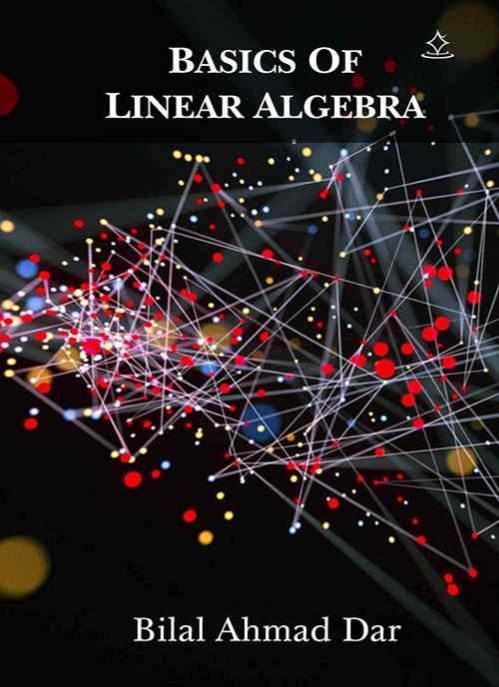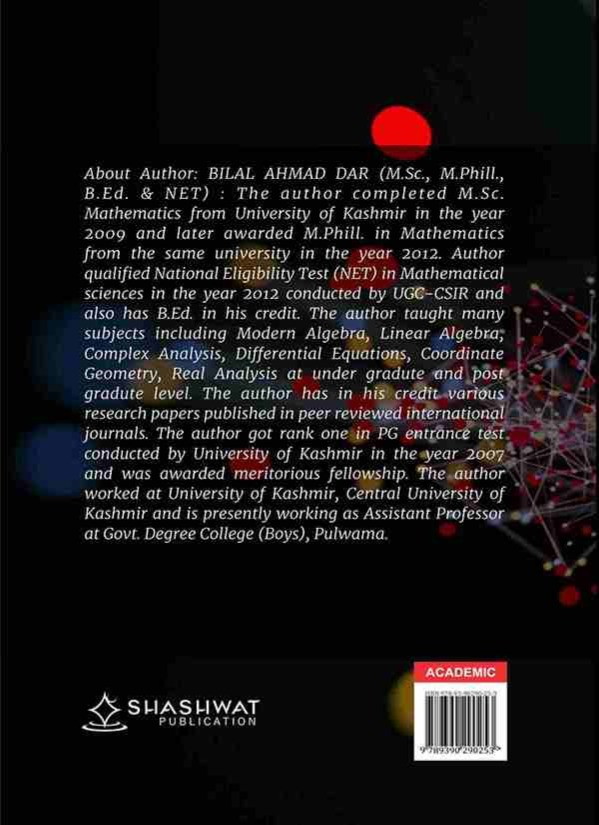### BASICS OF LINEAR ALGEBRA

NA
5.0

##### PAPERBACK Price : 450.00

About author : About Author: BILAL AHMAD DAR (M.Sc., M.Phill., B.Ed. & NET):: The author completed M.Sc. Mathematics from University of Kashmir in the year 2009 and later awarded M.Phill. in Mathematics from the same university in the year 2012. Author qualified National Eligibility Test (NET) in Mathematical sciences in the year 2012 conducted by UGC-CSIR and also has B.Ed. in his credit. The author taught many subjects including Modern Algebra, Linear Algebra, Complex Analysis, Differential Equations, Coordinate Geometry, Real Analysis at under gradute and post gradute level. The author has in his credit various research papers published in peer reviewed international journals. The author got rank one in PG entrance test conducted by University of Kashmir in the year 2007 and was awarded meritorious fellowship. The author worked at University of Kashmir, Central University of Kashmir and is presently working as Assistant Professor at Govt. Degree College (Boys), Pulwama.

About book : This book intends to develop a sense of understanding towards Linear Algebra. It will introduce a beginner to the basic fundamentals of linear algebra and their properties. The definitions are explained thoroughly and for better understanding various examples have been put forth for each definition. For the practice of students, some examples and results have been kept in each chapter. Important points deduced from theorems are written as remarks for the benefit of students. This book is different from other books because of two main reasons. First, the book contains various solved examples which makes the particular topic more understandable. Second, a number of multiple choice questions/objectives with answer keys are kept for each chapter which will help the students to qualify various competitive examinations. The book consists of six chapters. The first chapter gives a brief introduction of matrices wherein various types of matrices with examples are entioned. Also, the concept of determinants and adjoint of a matrix are explained briefly along with their properties. The second chapter deals with rank of a matrix, elementary transformations and elementary matrices. An important concept Echelon form of a matrix is mentioned and a method is introduced which explains how to determine rank of a matrix of any order. Third chapter deals with the linear dependence and independence of columns of a matrix and the behavior of matrix equation A X  O . A method is explained which tells how rank of a matrix gives information about the solution of Homogenous and Non-homogenous system of linear equations. Fourth chapter welcomes us with the central concept of linear algebra viz; Eigen values and Eigen vectors of a matrix. Many examples are solved which explains how many linearly independent Eigen vectors exist corresponding to an Eigen value and how to find them all.

## Customer ReviewsRs. 149.00